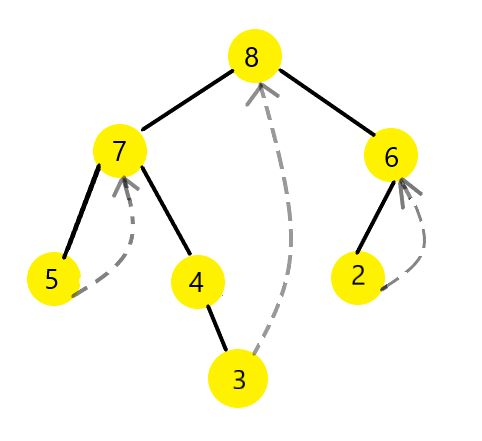×

Search anything:

# Morris Inorder Traversal in Binary Tree

#### Algorithms Problems on Binary TreeIn this article, you will learn about a method to traverse a tree in O(1) space complexity i.e without using recursion or stack. We will use the concept of Single Threaded Binary Tree.

2. Morris Inorder Traversal Algorithm
3. Morris Inorder Traversal Implementation in C++
4. Time and Space Complexity
5. Application of Morris Inorder Traversal
6. Conclusion

### 1. Single threaded binary tree

In single threaded binary trees,the right child pointers that are set to NULL are made to point to its inorder successor.

### 2. Morris Inorder Traversal Algorithm

We use the concept of single threaded binary tree for Morris Inorder Traversal.

While we traverse, if the current node in the tree has a left child or subtree, we trace its predecessor (in the inorder traversal) using an inner loop and set the right child of its predecessor to itself.

An indication that the left subtree of the current node has been traversed is when it reaches itself in the inner loop. We simply print this node, restore the right child of its predecessor to NULL and then traverse the right subtree.

If the current node does not have a left child or subtree, we simply print it and traverse the right subtree.

The algorithm is such that the initial state of the tree is restored after traversing through all the "predecessor" nodes.

### Pseudo code

``````curr = root
while(curr!=NULL):
if(curr->left==NULL):
print curr->val
curr= curr->right
else:
pred= findPredecessor(curr)
if(pred->right==NULL):
pred->right=curr
curr=curr->left
else:
pred->right=NULL
print curr->val
curr=curr->right
``````1. We initialize curr to the root of the tree. In this example, the root of the tree is node with value 8.

2. We check if curr->left==NULL. Since curr->left !=NULL, we find the predecessor of the curr node which is node 3.

3. We check if the predecessor node's right child is set to NULL. As node 3's right child is NULL, we initialize the right child of node 3 to curr node which is node 8.
We then set curr=curr->left which is node 7.

4. Since curr node 7's left child is not NULL, we find the predecessor of 7 which is 5.

5. Since 5's right child to curr node 7. We set curr= curr->left which is node 5.

6. Since curr node 5's left child is NULL, we print 5 and set curr= curr->right which is node 7.

7. Since curr node 7's left child is not NULL, we find its predecessor which is again, 5. We restore the tree by setting predecessor->right to NULL, i.e we remove the thread. We print 7 and then set curr= curr->right which is node 4.

8. Since curr node 4's left child is NULL, we print 4 and set curr=curr->right which is node 3.

9. Since curr node 3's left child is NULL, we print 3 and set curr= curr->right which is node 8 as found in step 3.

10. Since curr node 8's left child is not NULL, we find the predecessor which is again, 3. We restore the tree by setting predecessor->right to NULL, i.e we remove the thread. We print 8 and then set curr= curr->right which is node 6.

11. Since curr node 6's left child is not NULL, we find the predecessor of 6 which is node 2.

12. We set 2's right child to curr node 6 and curr=curr->left which is node 2.

13. Since curr node 2's left child is NULL, we print 2 and set curr= curr->right which is node 6.

14. Since curr node 6's left child is not NULL, we find the predecessor which is again, 2. We restore the tree by setting predecessor->right to NULL, i.e we remove the thread. We print 6 and then set curr= curr->right which is NULL.

15. Since curr==NULL, the while loop terminates.

### 3. Morris Inorder Traversal Implementation in C++

``````#include <bits/stdc++.h>

using namespace std;

class Tree {
public:
int val;
Tree* left;
Tree* right;
Tree(int x)
{
val=x;
left=right=NULL;
}
};

void Morris_inorderT(Tree* root)
{
Tree *curr, *pred;
curr = root;
while (curr != NULL)
{
if (curr->left == NULL) {
cout<<curr->val<<" ";
curr = curr->right;
}
else {
//finding predecessor
pred = curr->left;
while (pred->right&&pred->right != curr)
pred = pred->right;

if (pred->right == NULL)
{
pred->right = curr;
curr = curr->left;
}

else
{
pred->right = NULL;
cout<<curr->val<<" ";
curr = curr->right;
}
}
}
}

int main()
{
Tree* root = new Tree(8);
root->left = new Tree(7);
root->right = new Tree(6);
root->left->left = new Tree(5);
root->left->right = new Tree(4);
root->left->right->right = new Tree(3);
root->right->left = new Tree(2);

Morris_inorderT(root);

return 0;
}

``````

#### Output

``````5 7 4 3 8 2 6
``````

### 4. Time and Space Complexity

A binary tree with n nodes has n-1 edges. Each edge is visited at most 3 times - to visit, to find its predecessor if it exists, and to restore the right child of the predecessor to NULL.

Unlike using the methods such as recursion and stack for traversal, the auxiliary space for Morris Inorder Traversal = O(1) as we do not need to store the nodes or make recursive function calls.

### 5. Application of Morris Inorder Traversal

Morris Traversal can be used for inorder, preorder as well as postorder traversal without any auxiliary space.

### 6. Conclusion

With this article at OpenGenus, you now have a complete understanding of the Morris Inorder Traversal Technique to traverse a tree.